Next: Mach Number Up: One-Dimensional Compressible Inviscid Flow Previous: Sound Waves

# Bernoulli's Theorem

According to Bernoulli's theorem, which was introduced in Section 4.3, the quantity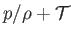is constant along a streamline in steady inviscid flow, where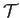is the total energy per unit mass. For the case of a compressible fluid,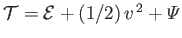. Hence, we deduce that(14.53)

is constant along a streamline. In particular, for an ideal gas, we find that(14.54)

is constant along a streamline, where use has been made of Equation (14.18).

Consider a solid object moving through an ideal gas. Generally speaking, there is at least one stagnation point in front of the object, where the gas comes to rest relative to it. At this point, the gas is adiabatically compressed, and there is an associated rise in temperature. In the frame of reference in which the object is at rest, and the gas a long way from it moves with constant speed and temperature, application of Bernoulli's theorem yields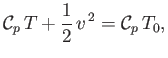(14.55)

where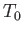is the stagnation-point temperature, and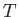the temperature at some general point where the flow speed is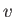. Thus, the total temperature rise due to adiabatic compression is(14.56)

whereandare the asymptotic flow speed and temperature, respectively. It can be seen that the stagnation temperature rise only depends on the velocity difference between the object and the gas, and is independent of the gas's density, temperature, or pressure. Note, however, that a lower molar mass implies a higher specific heat, and, hence, a smaller stagnation temperature rise.Next: Mach Number Up: One-Dimensional Compressible Inviscid Flow Previous: Sound Waves
Richard Fitzpatrick 2016-03-31